﻿ Rotational Displacement Analysis of PlateExamples | Product | Murata Software Co., Ltd.Example55Rotational Displacement Analysis of Plate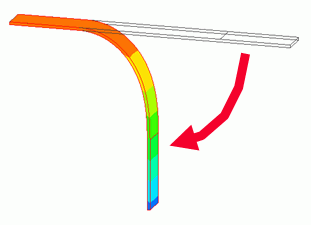General

• A plate bent in 90-degree angle is analyzed.

• Analysis results will be compared where the option of [Rotate at constant radius] is selected and deselected for the Rotational displacement.

• Unless specified in the list below, the default conditions will be applied.

Analysis Space

 Item Settings Analysis Space 3D Model unit mm

Analysis Conditions

Select [Large displacement] in the Large Deformation as deformation of 90° is large.

 Item Setting Solver Mechanical Stress Analysis [Galileo] Analysis Type Static analysis Large Deformation Select Large displacement

The Step/Thermal Load tab is set as follows.

 Tab Setting Item Setting Step/Thermal Load Options for the Multi-Step Analysis Save the results of substeps: Deselect

Graphical Objects

The model is a box solid body with edge length of 200mm. The material is iron.

Fix the left end in the X direction. Fix the 50mm area of the left end at its bottom in the Z direction.
The plate is bent at its center in 90-degree angle. Set the rotational displacement on the 50mm area of the right end at its top side.

Set the general mesh size at 2.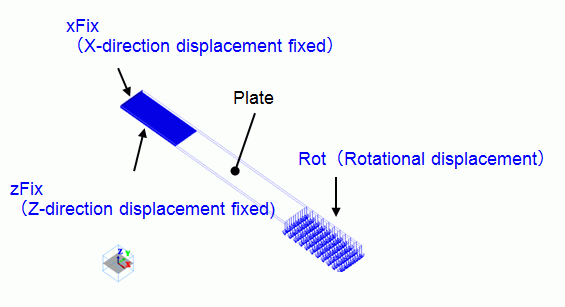Body Attributes and Materials

 Body Number/Type Body Attribute Name Material Name 0/Solid PLATE 007_Fe *1) 1,2/Face No setup *2) No setup *2)

*Note 1) Select from the Material DB.

*Note 2) Imprinting body for the boundary condition.

Boundary Condition

 Boundary Condition Name/Topology Tab Boundary Condition Type Settings xFix/Face Mechanical Displacement Select the X Component. UX=0 zFix/Face Mechanical Displacement Select the Z Component. UZ=0 Rot/Face Mechanical Rotational displacement Coordinates on the Axis (100, 0.0, 2) Vectors of the Axis (0.0, 1, 0.0) Rotation Angle 90[deg] Rotate at constant radius Model 1: Deselect, Model 2: Select

Results

The deformation of the Model 1 is shown below. The contour diagrams show displacement in X direction and Z direction respectively.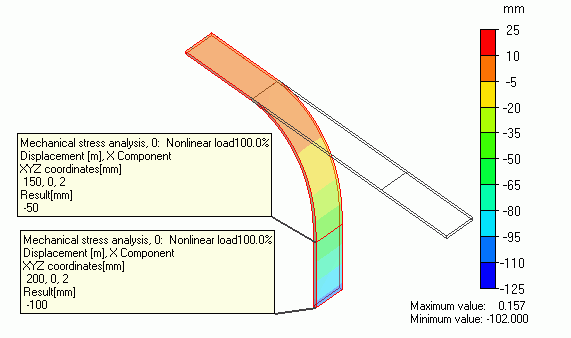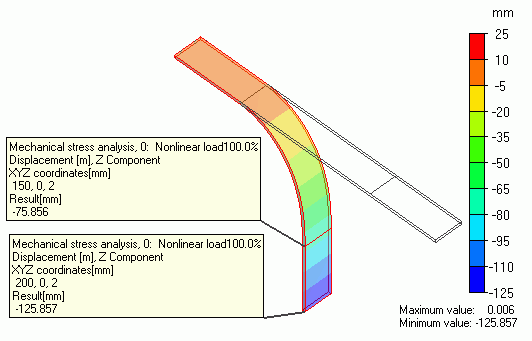The displacement at the end and middle of the plate shows
-50 and -100 in the X direction as defined.

The displacement in the Z direction shows -75.856 and -125.857.

The results are larger than -50, and -100 in the case where the radius is constant. It indicates the radius changed during the rotation.

The reactive force at the boundary condition [Rot] in the table shows

almost 0 in the Y and Z directions and -798[N] in the X direction only.

If [Rotate at constant radius] is deselected, the force will be applied in the rotational direction during the rotation.

The deformation of the Model 2 is shown below. The contour diagrams below show displacement in X direction and Z direction respectively.

Unlike Model 1, the plate is bent in a complex way.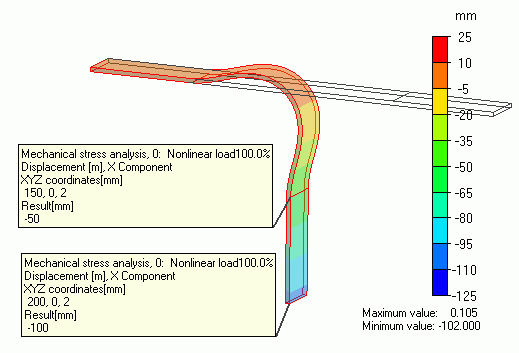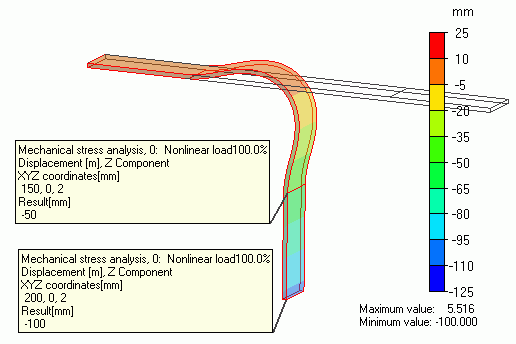The displacement at the end and middle of the plate shows
-50 and -100 in the X direction as defined.

The displacement in the Z direction also shows -50 and -100.

It indicates the model is rotating at the constant radius.

The reactive force at the boundary condition [Rot] in the table shows

almost 0 in the Y direction and -4759[N] in the X direction and 4727[N] in the Z direction.

Unlike the Model 1, large force is taking place in the Z direction too.

If [Rotate at constant radius] is selected, a force is required to keep the radius constant.
Therefore, the force in Z direction is generated.

﻿